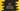# C++ program to find the square root of a numberIn this post, I will talk about how to find the square root of a number in C. A number x is called square root of y if we multiply x with x, we will get y, i.e. xx = y. For example, square root of *16 is 4.

For finding out the square root, we can use pow() and sqrt() methods defined in the cmath library. These methods makes it easier to find tthe square root. **Let me show you how :

## Method 1: Find square root using pow() in C++ :

pow() method is used to find the power of a number. It takes two arguments : the first one is the number itself and the second one is the power value. To find the square root of a number using pow(), we can pass 0.5 or 1/2. That will give us the square root.

### C++ program :

``````#include <iostream>
#include <cmath>
using namespace std;

int main()
{
int givenNumber, squareRoot;

cout << "Enter a number : " << endl;
cin >> givenNumber;

cout << "Square root of " << givenNumber << " is: " << pow(givenNumber, .5) << endl;

return 0;
}``````

In this example, we are taking the number as input from the user and storing it in the variable givenNumber. Next, we are using pow() to find the power of that user input number.

### Sample Output :

``````Enter a number :
16
Square root of 16 is: 4

Enter a number :
100
Square root of 100 is: 10``````

## Method 2: Find square root using sqrt() in C++ :

sqrt is a straight forward method. It is used to find the square root of a number. It takes the number as its argument and returns the square root. This method is also defined in cmath.

``````#include <iostream>
#include <cmath>
using namespace std;

int main()
{
int givenNumber, squareRoot;

cout << "Enter a number : " << endl;
cin >> givenNumber;

cout << "Square root of " << givenNumber << " is: " << sqrt(givenNumber) << endl;

return 0;
}``````

### Sample Output :

``````Enter a number :
144
Square root of 144 is: 12
(base) ➜  c++ ./example
Enter a number :
12345
Square root of 12345 is: 111.108``````